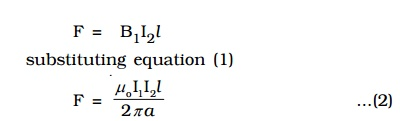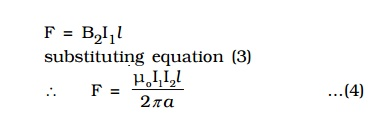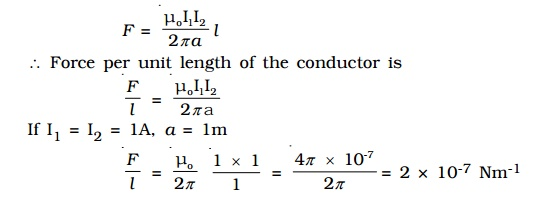Home | | Physics | Force on a current carrying conductor placed in a magnetic field

# Force on a current carrying conductor placed in a magnetic field

Let us consider a conductor PQ of length l and area of cross section A.

Force on a current carrying conductor placed in a magnetic field.

Let us consider a conductor PQ of length l and area of cross section A. The conductor is placed in a uniform magnetic field of induction B making an angle θ with the field [Fig 3.22].A current I flows along PQ. Hence, the electrons are drifted along QP with drift velocity vd. If n is the number of free electrons per unit volume in the conductor, then the current is

I = nAvdeThe negative sign in the equation indicates that the direction of current is opposite to the direction of drift velocity of the electrons.

Since the electrons move under the influence of magnetic field, the magnetic lorentz force on a moving electron.This total force on all the moving free electrons is the force on the current carrying conductor placed in the magnetic field.

## Magnitude of the force

The magnitude of the force is    F = BIl sin θ

1.     If the conductor is placed along the direction of the magnetic field, θ = 0o, Therefore force F = 0.

2.     If the conductor is placed perpendicular to the magnetic field, = 90o, F = BIl. Therefore the conductor experiences maximum force.

## Direction of force

The direction of the force on a current carrying conductor placed in a magnetic field is given by Fleming’s Left Hand Rule.

The forefinger, the middle finger and the thumb of the left hand are stretched in mutually perpendicular directions. If the forefinger points in the direction of the magnetic field, the middle finger points in the direction of the current, then the thumb points in the direction of the force on the conductor.

## 1 Force between two long parallel current-carrying conductors

AB and CD are two straight very long parallel conductors placed in air at a distance a. They carry currents I1 and I2 respectively. (Fig 3.23). The magnetic induction due to current I1 in AB at a distance a isThis magnetic field acts perpendicular to the plane of the paper and inwards. The conductor CD with current I2 is situated in this magnetic field. Hence, force on a segment of length l of CD due to magnetic field B1 isBy Fleming’s Left Hand Rule, F acts towards left. Similarly, the magnetic induction due to current I2 flowing in CD at a distance a isThis magnetic field acts perpendicular to the plane of the paper and outwards.

The conductor AB with current I1, is situated in this field. Hence force on a segment of length l of AB due to magnetic field B2 isBy Fleming’s left hand rule, this force acts towards right. These two forces given in equations (2) and (4) attract each other. Hence, two parallel wires carrying currents in the same direction attract each other and if they carry currents in the opposite direction, repel each other.

### Definition of ampere

The force between two parallel wires carrying currents on a segment of length l isThe above conditions lead the following definition of ampere.

Ampere is defined as that constant current which when flowing through two parallel infinitely long straight conductors of negligible cross section and placed in air or vacuum at a distance of one metre apart, experience a force of 2 × 10-7 newton per unit length of the conductor.

Study Material, Lecturing Notes, Assignment, Reference, Wiki description explanation, brief detail
Physics : Effects of electric current : Higher Secondary(12 Std) : Force on a current carrying conductor placed in a magnetic field |